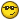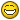# mathematics help

Hello I’m doing some matrix calculations in excel and i have a very easy question.

Which function to choose in excel to add two matrices.

Thanks,

JR(doing his homework)Thanks,

works great!!! but is ther also a way to do it without define a name first, so with a function??? statistic???

Thanks,

JRThat was me who replied to you but I forgot to loginTo add matrices just add them using Names of Ranges (A and B for example).
You can write a function in Excel VBA and call it MADD ( A , B ) for example.
This function defines a first range MxN (it can be a random range) and names it A, and a second range KxL and names it B. Then this function compares these ranges to make sure that M=K and N=L.
Then the function selects another range PxQ (P=M=K and Q=N=L) and applies to it:
Selection.FormulaArray = “=A+B”

Unlike Multiplication and finding inverses, there is no ready function to add as far as I know.

I never even knew Excel could do matrix calculations…
From where did you get this homework?

Many people with Excel do not appreciate or know that they actually have a complete development environmentIf you know Excel VBA, you can do whatever you like either by writing functions and procedures or by using Add-Ons. You can also communicate with other programs using the Win32 COM extensions (for example you can totally automate Excel using Python). It is quite cool and powerful. And the only technology that Microsoft got quite right. I myself use Excel for example to calculate series of curved splined points to use in animations.

Thanks,I know how to do it in Maple but was curious if it was possible in excel, because i think excel in better for complicated calculations

JR@martinal

I study chemistry in holland. We also use Maple. We use maple and excel to do chemical calculations.

JRHope this was of some helpand good luck with your work!

For complicated (numerical) matrix calculations matlab is excellent. It’s becoming the most used mathprogram in the University of Oslo where I study, since it eats Maple for breakfast when it comes to numerical vector and matrixbased calculations@lunar07 : Thanks

@martinal:
mmmmm mayby i’ll have to try mathlab. Thanks for the tip.

JRIt’s “matlab”, not “mathlab”. I think the name comes from Matrix not Math.

mmmmmmmmmmmmmmThanks

JR